# RC differentiator Circuit Design

### RC differentiator:

RC differentiator is a series connecting RC network. Its output use to the math processes. The RC differentiator is a capacitance in series with a resistance. It is a frequency-dependent device which has reactance in series with a fixed resistance. Just like the integrator circuit, the output voltage depends on the circuits RC time constant and input frequency.

### RC differentiator Circuit Design:

A circuit in which output voltage is directly proportional to the derivative of the input  is known as a differentiating circuit.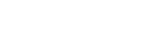A differentiating circuit is a simple series RC circuit where the output is taken across the resistor R.

The circuit is suitably designed so that the output is proportional to the derivative of the input.

Thus if a d.c. or constant input is applied to such a circuit, the output will be zero.Fig.1 shows a typical differentiating circuit.

In order to achieve good differentiation, the following two conditions should be satisfied:

1. The time constant RC of the circuit should be much smaller than the time period of the input wave.
2. The value of XC should be 10 or more times larger than R at the operating frequency.

Fulfilled these conditions, the output across R will be the derivative of the input.

Let ei be the input alternating voltage

And let  i be the resulting alternating current.

The charge q on the capacitor  C at any instant is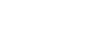Now,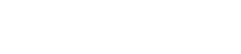Or,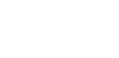Since the capacities reactance is very much larger than R, the input voltage can be considered equal to the capacitor voltage with negligible error i.e.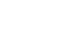So,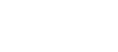Output voltage,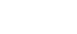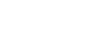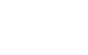Hence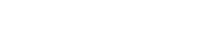### Input Square Wave:

When the input fed to a differentiating circuit is a square wave, output will consist of sharp narrow pulses as shown in Fig.2.Fig.2

During the part of input square wave, its amplitude changes abruptly and hence the differentiated wave will be a sharp narrow pulse as shown in fig.2.

However, during the constant part CB of the input, the output will be zero because the derivative of a constant is zero.

Let us look at the physical explanation of this behavior of the circuit.

Since time constant RC of the circuit is very small w.r.t. time period of input wave and XC>>R,

The capacitor will become fully charged during the early part of each half-cycle of the input wave.

During the remainder part of the half-cycle, the output of the circuit will be zero because the capacitor voltage (ec) neutralizes the input voltage and there can be no current flow through R.

Thus we shall get sharp pulse at the output during the start of each half-cycle of input wave while for the remainder part of the half-cycle of input wave, the output will be zero.

Such pulses are used in many ways in electronics circuits e.g. in television transmitters and receivers, in multi vibrators to initiate action etc.

### RC Waveform Output:Fig 4: RC Waveform Output (Differentiate)

So by having an RC value of one tenth the pulse width (and in our example above this is 0.1 x 10mS = 1mS) or lower we can produce the required spikes at the output, and the lower the RC time constant for a given pulse width, the sharper the spikes. Thus the exact shape of the output waveform depends on the value of the RC time constant.

### Conclusion:

We have seen here in this RC Differentiator tutorial that the input signal is applied to one side of a capacitor and the the output is taken across the resistor. A differentiator circuit is used to produce trigger or spiked typed pulses for timing circuit applications.

When a square wave step input is applied to this RC circuit, it produces a completely different wave shape at the output. The shape of the output waveform depending on the periodic time, T (an therefore the frequency, ƒ) of the input square wave and on the circuit’s RC time constant value.

When the periodic time of the input waveform is similar too, or shorter than, (higher frequency) the circuits RC time constant, the output waveform resembles the input waveform, that is a square wave profile. When the periodic time of the input waveform is much longer than, (lower frequency) the circuits RC time constant, the output waveform resembles narrow positive and negative spikes.

The positive spike at the output is produced by the leading-edge of the input square wave, while the negative spike at the output is produced by the falling-edge of the input square wave. Then the output of an RC differentiator circuit depends on the rate of change of the input voltage as the effect is very similar to the mathematical function of differentiation.

Live Simulation: RC differentiator Circuit

See My All Simulation